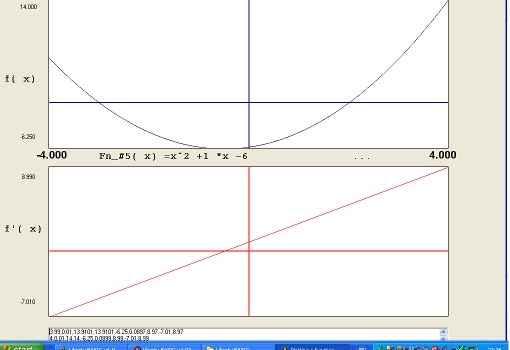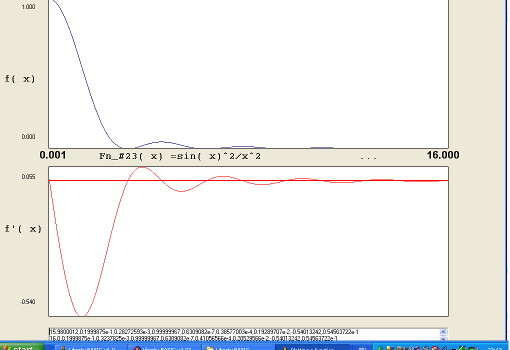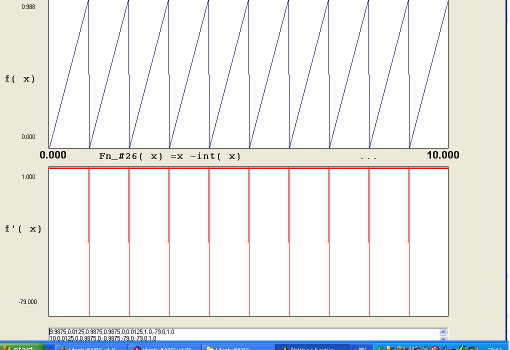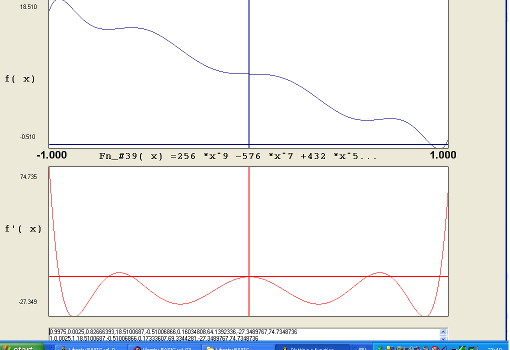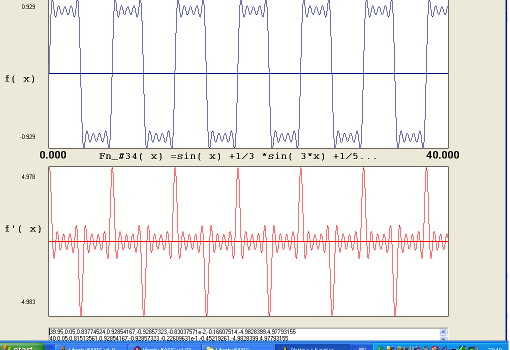# Graphical display of functions of x and their gradients.

NB This is an unfinished program. It will be updated as and when I make changes!

This program takes f( x), expressed in BASIC syntax, and plots it and its gradient f'(x) over a chosen range of x. It autoscales so the max & minimum f( x) and f'(x) to fit their graphic area limits. If x-axis or y-axis are in-range, they are plotted, but no oither gridlines are shown. If the limits or function are badly-chosen or pathological in some way, it may fail with 'divide-by-zero' or infinity limits problems. If f'( x) or f'(x) are constant, that is declared.
If you know the analytic form of f'(x) (ie can differentiate f(x) wrt x) then you can confirm the results. Even if you cannot, you should be able to see how gradients of f(x) are shown as values of f'(x).I would be interested in comments and ideas. Mail me at mr.john.f@gmail.com